Terms, Factors and Coefficients

Chapter 10 Class 7 Algebraic Expressions
Concept wise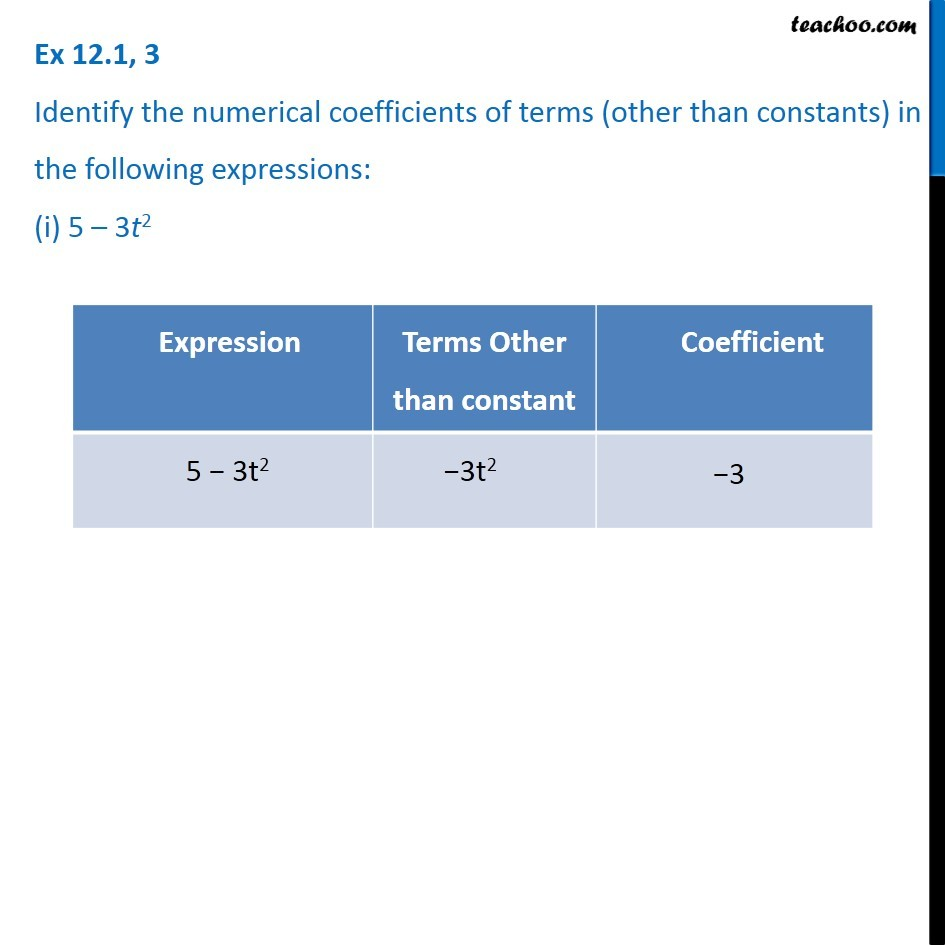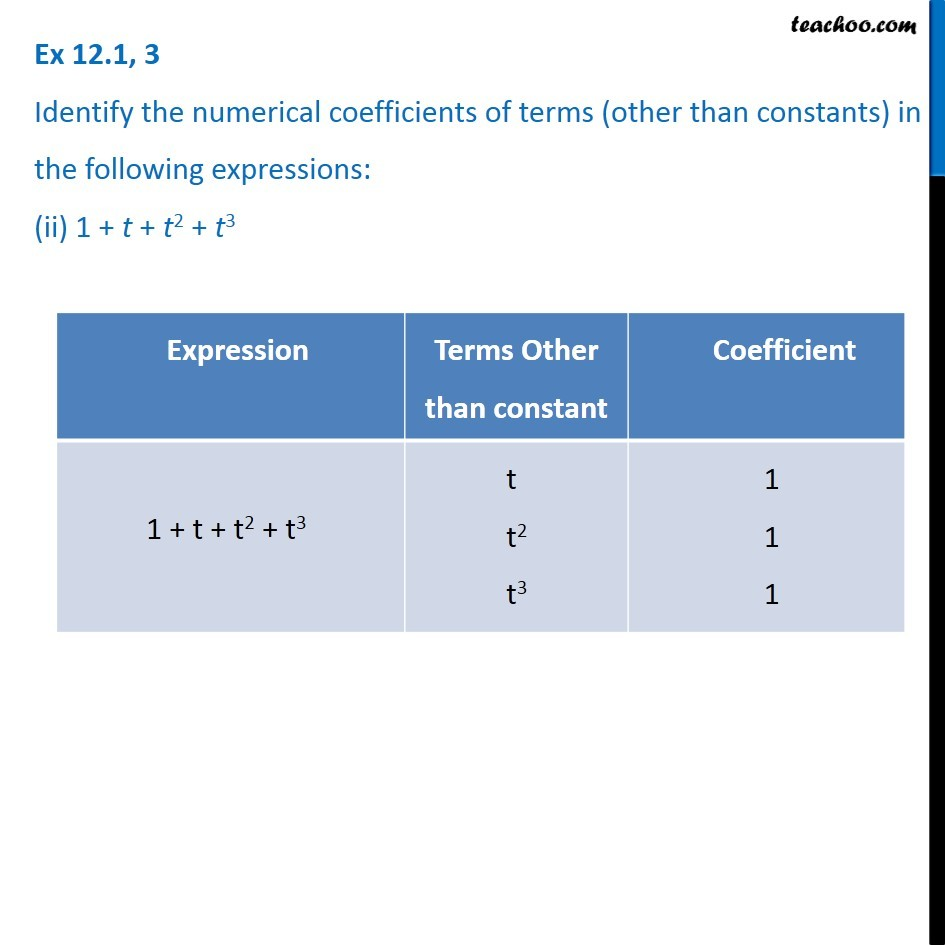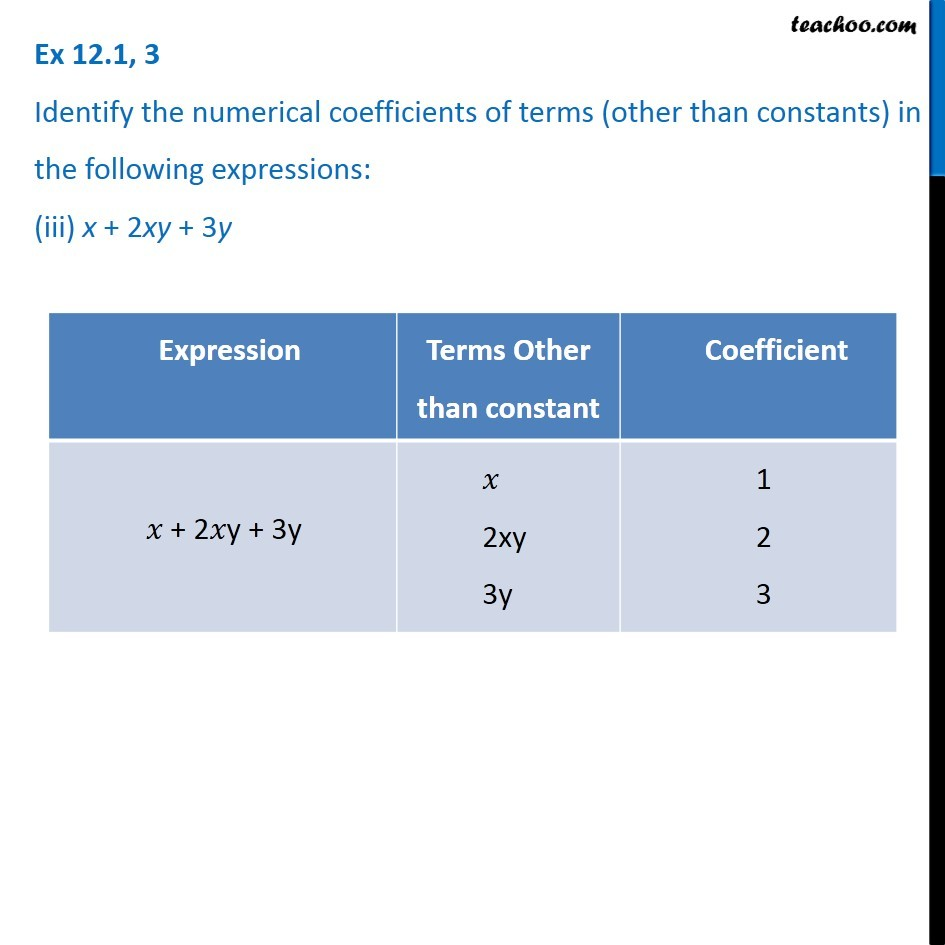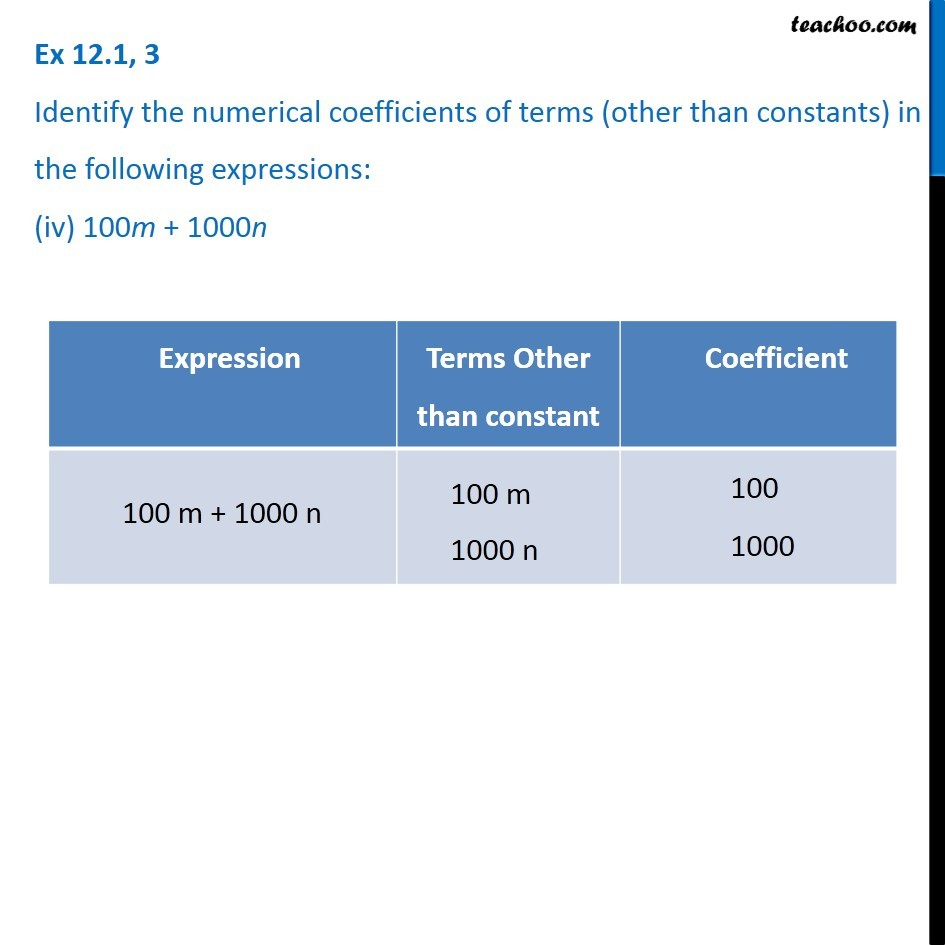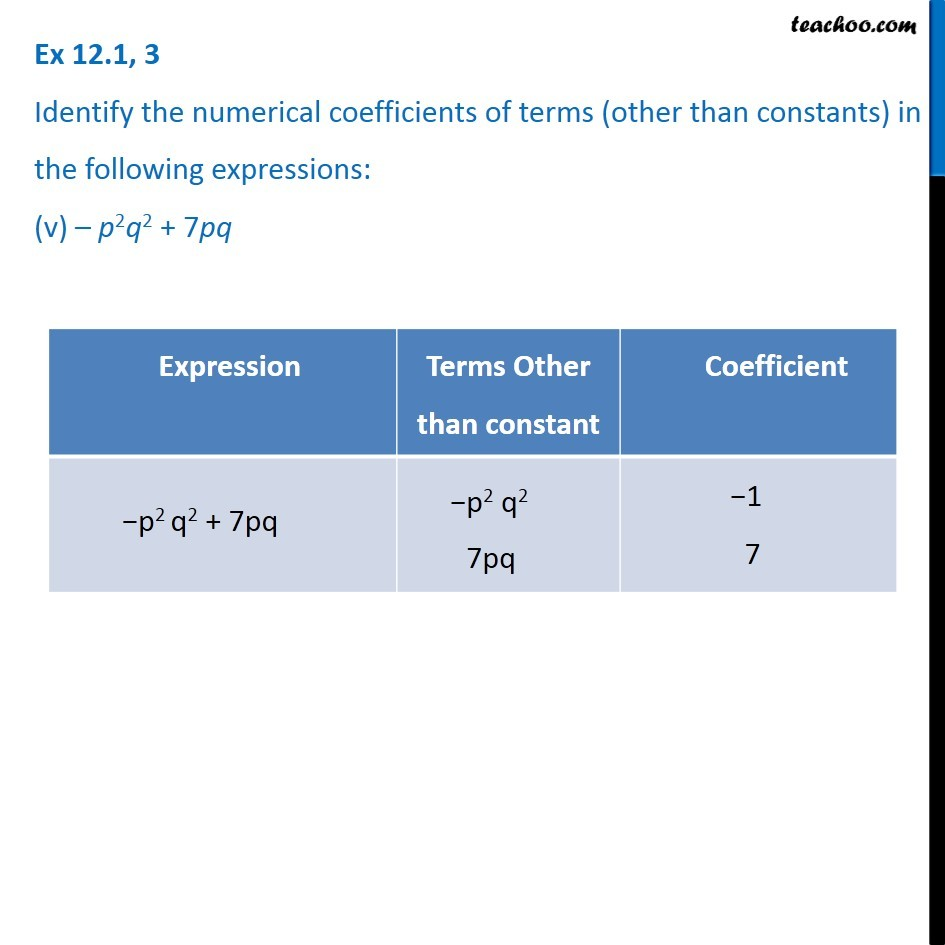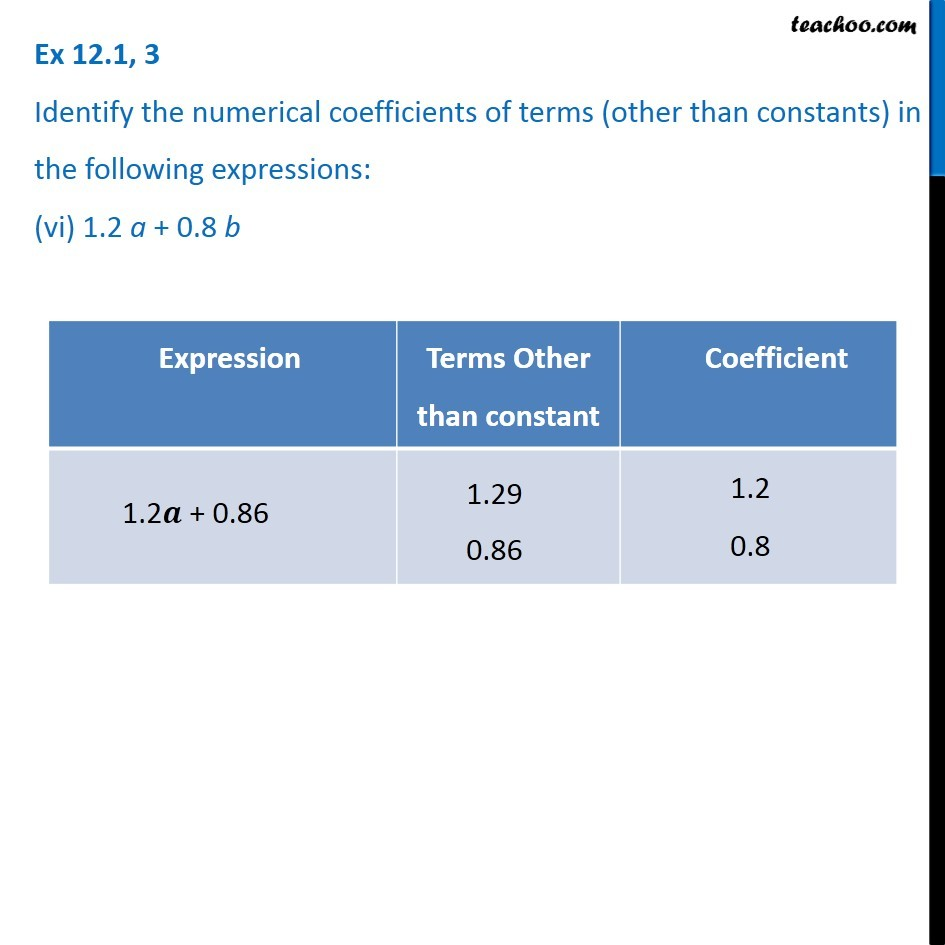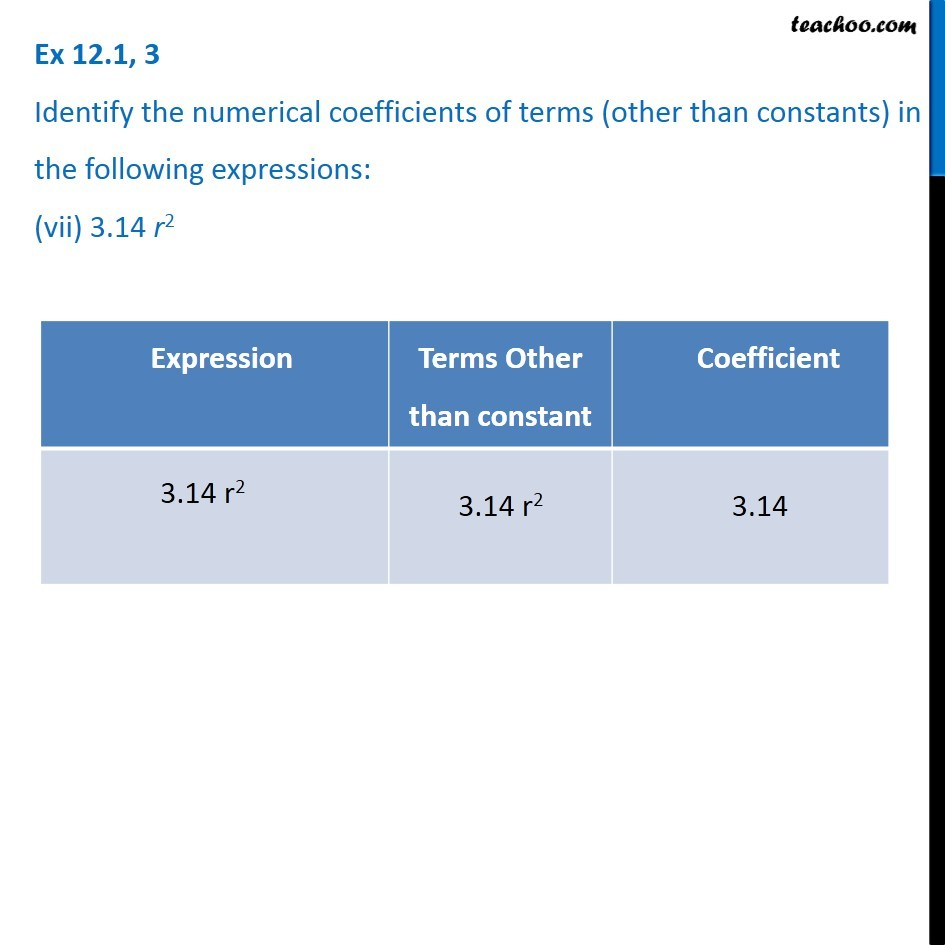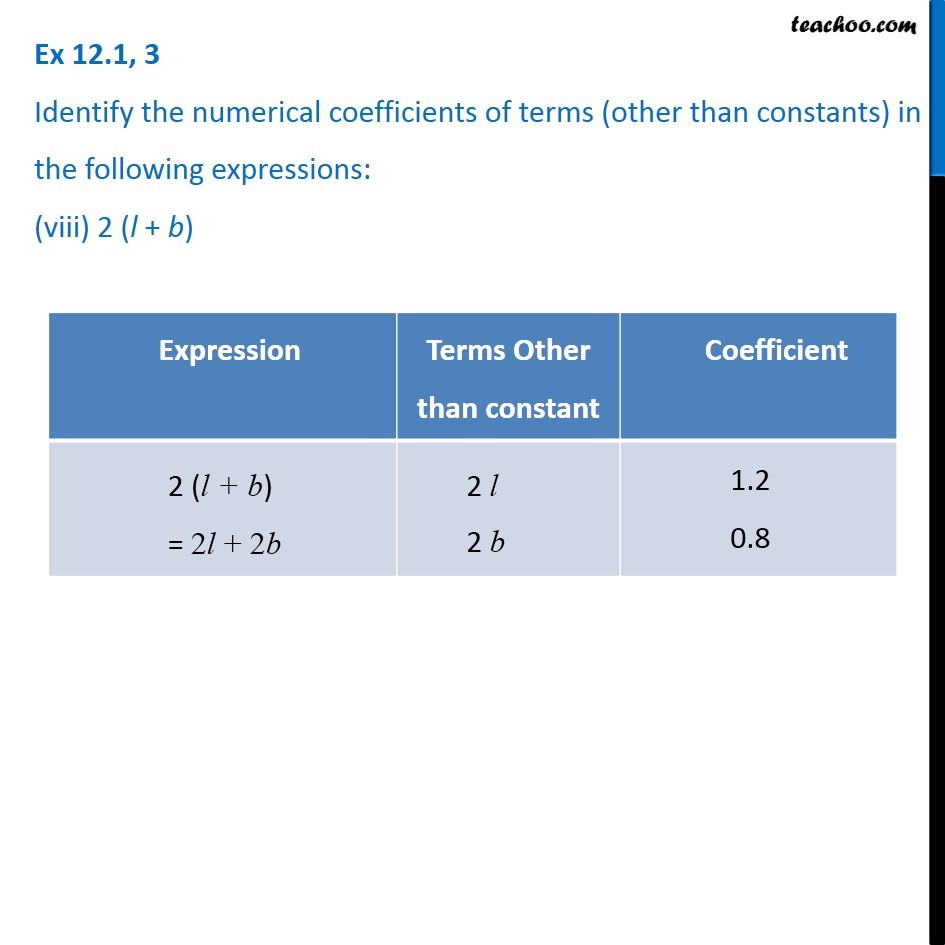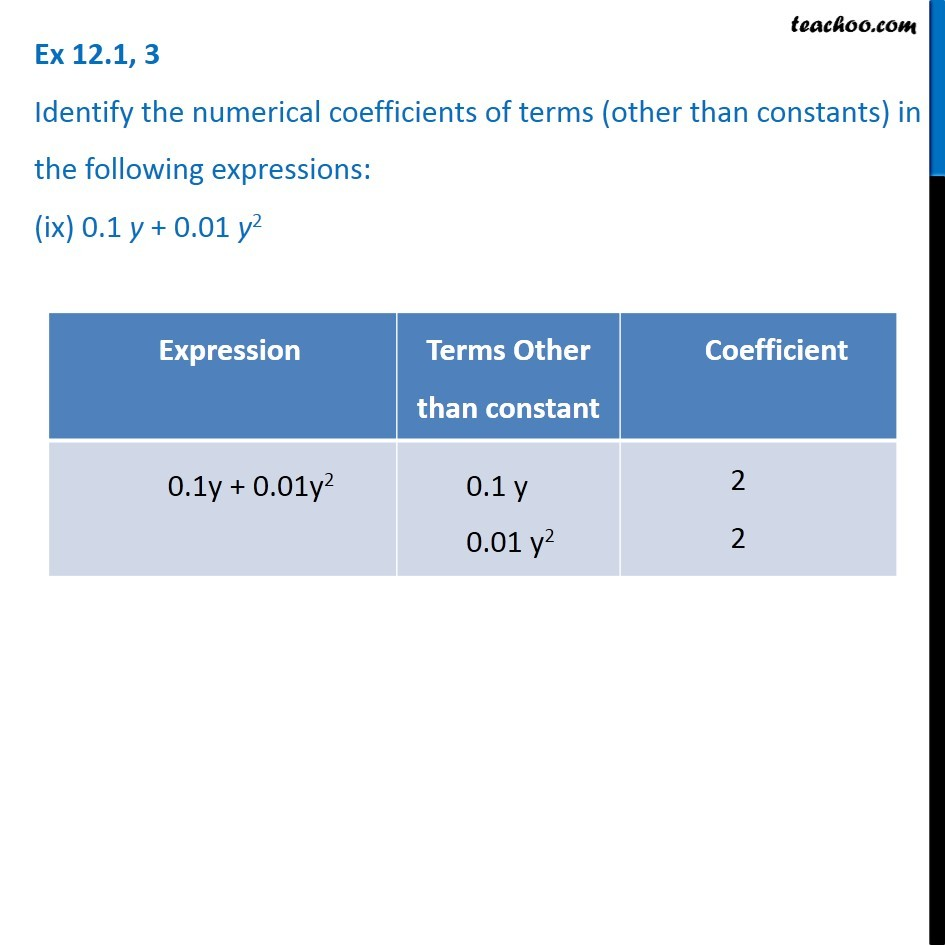Learn in your speed, with individual attention - Teachoo Maths 1-on-1 Class

### Transcript

Ex 10.1, 3 Identify the numerical coefficients of terms (other than constants) in the following expressions: (i) 5 – 3t2 Expression Terms Other than constant Coefficient 5 -- 3t2 --3t2 -3 Ex 10.1, 3 Identify the numerical coefficients of terms (other than constants) in the following expressions: (ii) 1 + t + t2 + t3 Ex 10.1, 3 Identify the numerical coefficients of terms (other than constants) in the following expressions: (iii) x + 2xy + 3y Ex 10.1, 3 Identify the numerical coefficients of terms (other than constants) in the following expressions: (iv) 100m + 1000n Ex 10.1, 3 Identify the numerical coefficients of terms (other than constants) in the following expressions: (v) – p2q2 + 7pq Ex 10.1, 3 Identify the numerical coefficients of terms (other than constants) in the following expressions: (vi) 1.2 a + 0.8 b Ex 10.1, 3 Identify the numerical coefficients of terms (other than constants) in the following expressions: (vii) 3.14 r2 Ex 10.1, 3 Identify the numerical coefficients of terms (other than constants) in the following expressions: (viii) 2 (l + b) Ex 10.1, 3 Identify the numerical coefficients of terms (other than constants) in the following expressions: (ix) 0.1 y + 0.01 y2